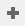# Calculating the time in minutes between two time variables

With Snap Desktop you can use times to analyze your survey response data. This tutorial explains how to set up the start and end time questions then create variables that calculate the time difference and show how to categorise this information as time bands.

## Step 1: Creating the time questions

1. In the Questionnaire window, create two open ended questions. These are used to enter the start time and end time.
2. In the Topics toolbar select Response and set the question response type to Time.

## Step 2: Creating a variable to calculate the time difference

A Variable needs to be set up in order for Snap to calculate the interval between the Start and End time. This variable needs to be set up as a Derived, Quantity-Response Variable. That is, a new variable deriving (taking information) from other variables.

1. In the Variables window, click New Variableto create a new Variable.
2. Ensure the following settings are applied:
• Name: V1
• Label: Time difference
• Type: Derived
• Response: Quantity
3. In the Values column in the same row as Not Ask type: Q1 missing or Q2 missing. This will ensure that the time difference is not calculated if Q1 or Q2 are empty.
4. Type the formula below in the values column but this time in the same row as Valid:

((Q2\1)*60 + (Q2-Q2\1)*100) – ((Q1\1)*60 + (Q1-Q1\1)*100) + (1440 * num(Q2<Q1))

The formula is divided into 3 sections. The first section converts the answers to Q2 into minutes, the second section converts Q1 into minutes. The last section is only needed if times could span midnight – it adds 1440 minutes if Q2 has an answer after midnight and Q1 has an answer before midnight.

Note: Time is analysed in minutes. There are 60 minutes in each hour and 1440 minutes in 24 hours: 60 (minutes in an hour) X 24 (hours in a day)

## Step 3: Creating a derived variable to display time categories

Another variable needs to be set in order to display the results in time categories.

1. In the Variables window, click New Variableto create a new Variable.
2. Enter the following settings:
• Name: V2
• Label: Time categories
• Text: Duration between start and end times
• Type: Derived
• Response: Single
3. Add the following code information in the Code list to create the categories and values:
1. Click on the Count Responses button to calculate the responses. The Counts column in the variable will display the results.
2. Click on the Save button. You can use the variable to create a table or chart of the results by entering the derived variable name in the analysis box of your table or chart to generate the results.

## Using other built-in time functions

Other built-in functions that may be used for extracting information from times are shown in the table.

You can build a similar derived variable to categorize replies according to the day of week, regardless of the month or the year, by using the weekday function in a similar way to the way the month function was used in the previous example.

If there is a topic you would like a tutorial on, email to snapideas@snapsurveys.com

Contents